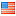This animation intends to show how four classic signals can be approximated with order limited Fourier series.
One can note that:
*) the approximation is as easier as the function (and its derivatives) is (are) continuous.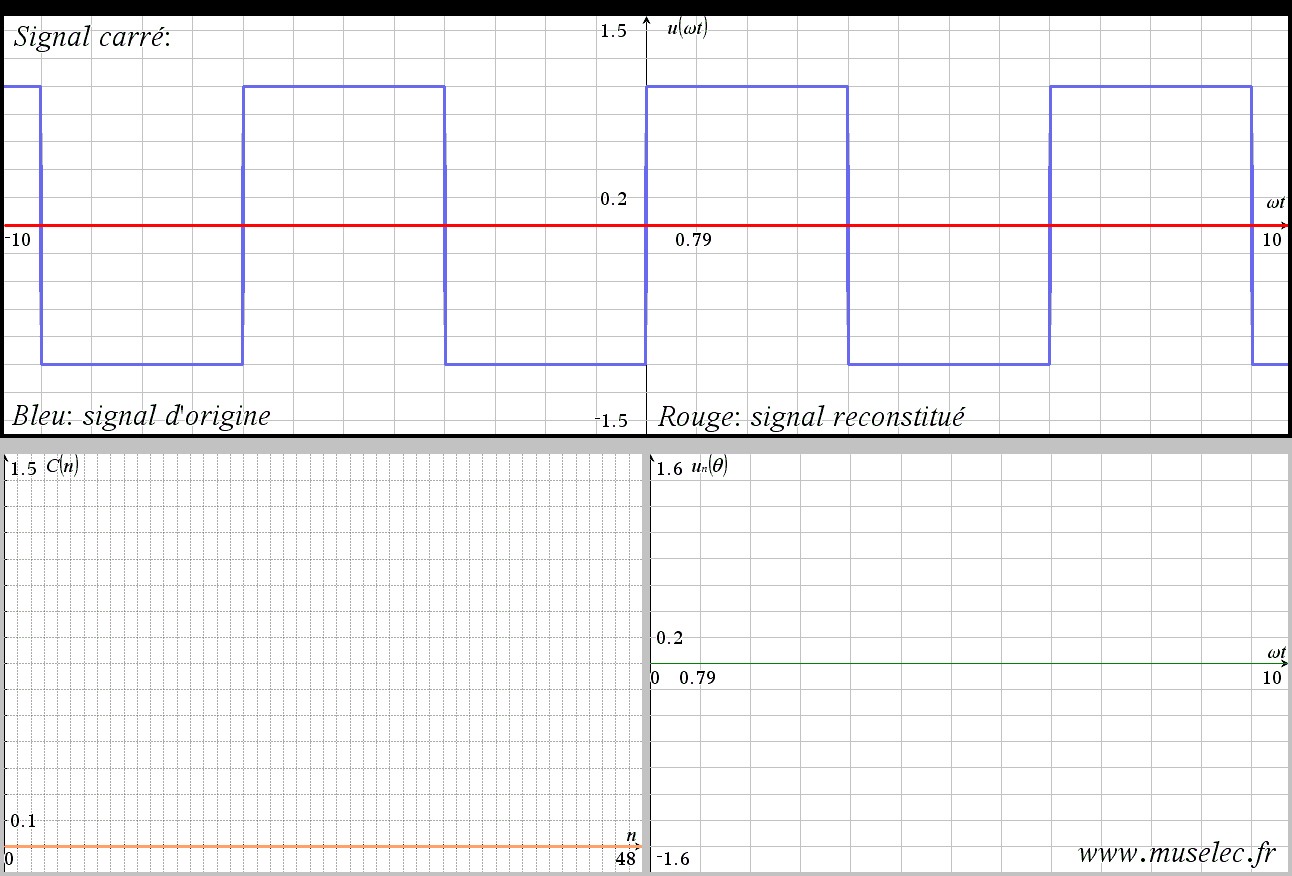Square wave and its harmonics.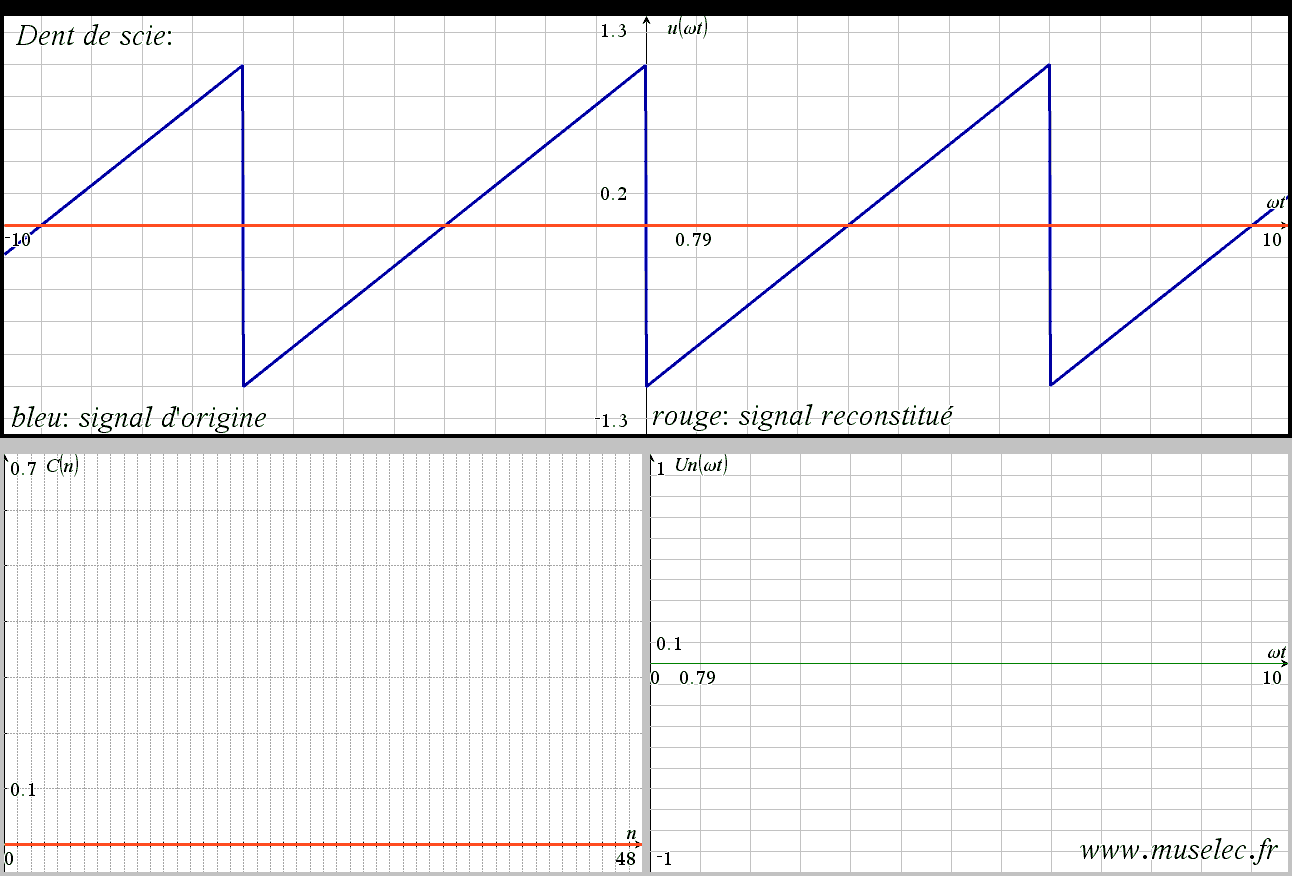Saw wave and its harmonics...Spectrum of a PWM signal...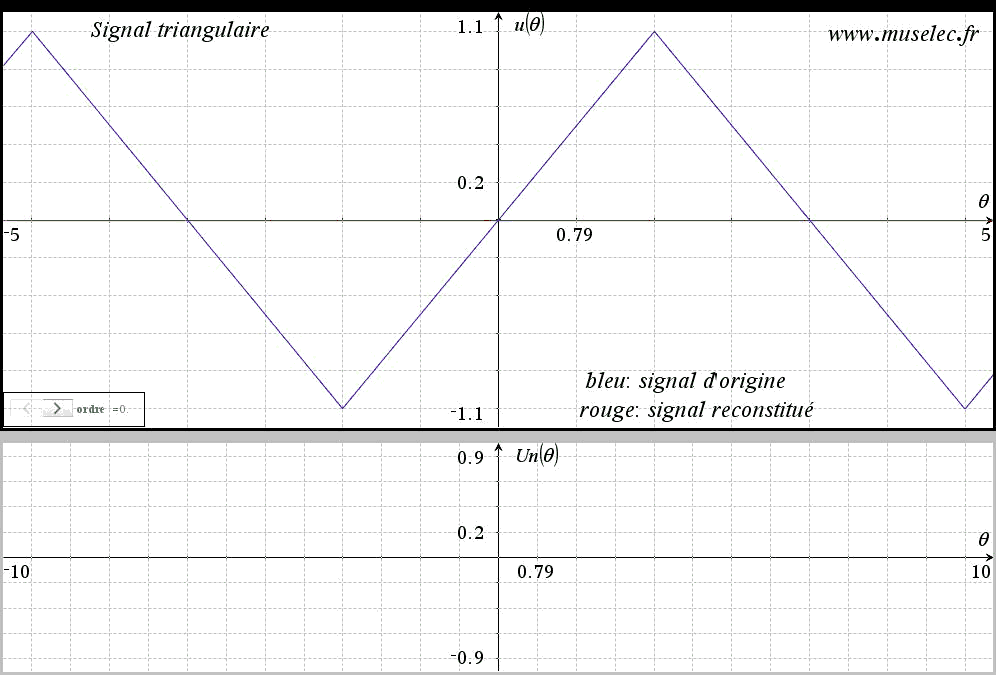Triangle wave and its harmonics...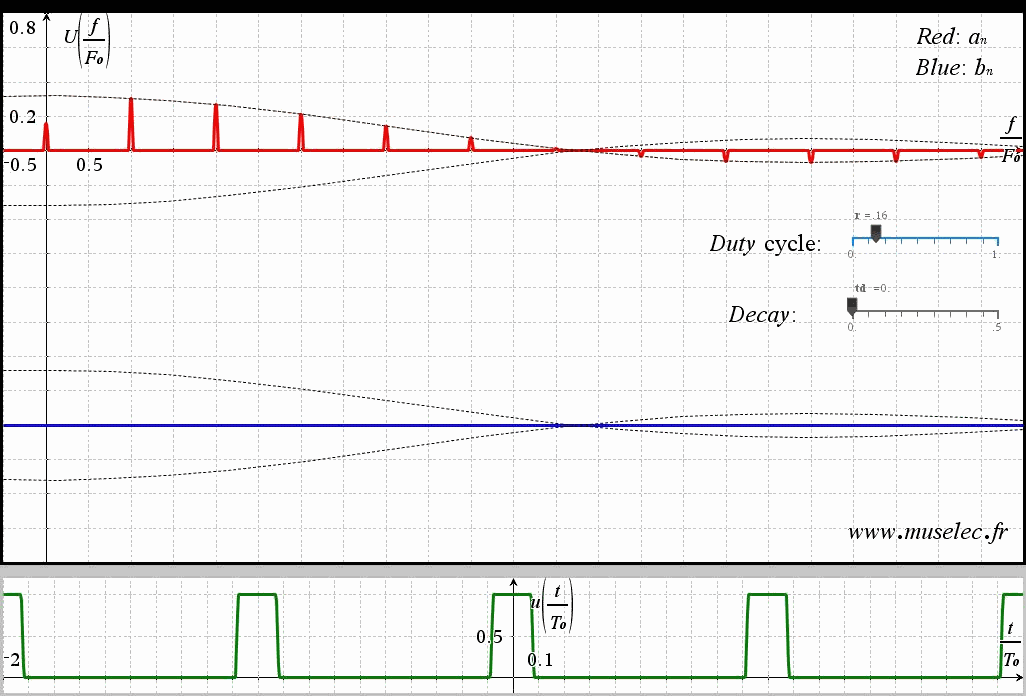This animation intends to show the relationship between the An and Bn coeffs and the cyle ratio and the decay of a PWM signal.This animation intends to show how to do the link between a Fourier series and a Fourier transform. The example is the one of a sine card. The sine card repeats itself periodicaly. The period grows and the limit is the Fourier transform when doing a density measure. Note that you have to use an adaptive density measure function (blue).
Also note that a sine card periodically repeated is a constant within a given period.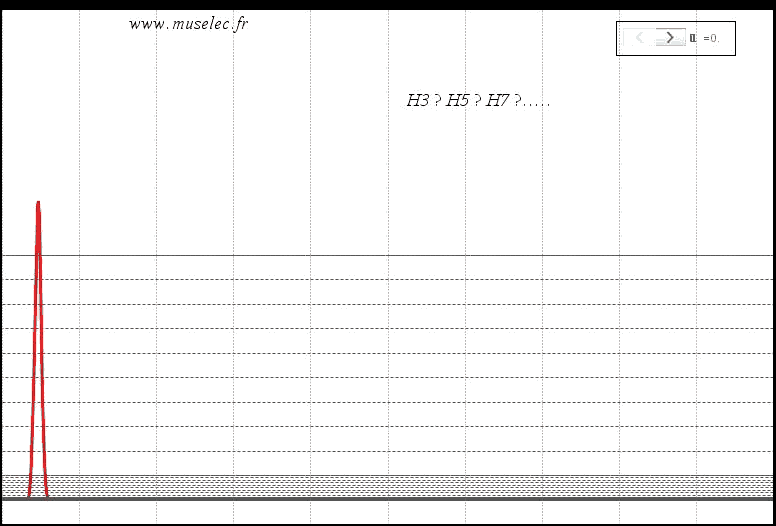Distorsion spectrum of a XR2201 sine wave. Lin to Log scale animation.